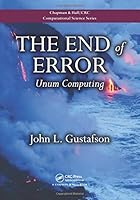# The End of Error: Unum Computing## Book Description

The Future of Numerical Computing

Written by one of the foremost experts in high-performance computing and the inventor of Gustafson’s Law, The End of Error: Unum Computing explains a new approach to computer arithmetic: the universal number (unum). The unum encompasses all IEEE floating-point formats as well as fixed-point and exact integer arithmetic. This new number type obtains more accurate answers than floating-point arithmetic yet uses fewer bits in many cases, saving memory, bandwidth, energy, and power.

A Complete Revamp of Computer Arithmetic from the Ground Up

Richly illustrated in color, this groundbreaking book represents a fundamental change in how to perform calculations automatically. It illustrates how this novel approach can solve problems that have vexed engineers and scientists for decades, including problems that have been historically limited to serial processing.

Suitable for Anyone Using Computers for Calculations

The book is accessible to anyone who uses computers for technical calculations, with much of the book only requiring high school math. The author makes the mathematics interesting through numerous analogies. He clearly defines jargon and uses color-coded boxes for mathematical formulas, computer code, important descriptions, and exercises.

Part 1 - A New Number Format: The Unum
Chapter 1 - Overview
Chapter 2 - Building up to the unum format
Chapter 3 - The “original sin” of computer arithmetic
Chapter 4 - The complete unum format
Chapter 5 - Hidden scratchpads and the three layers
Chapter 6 - Information per bit
Chapter 7 - Fixed-size unum storage
Chapter 8 - Comparison operations
Chapter 9 - Add/subtract and the unbiased rounding myth
Chapter 10 - Multiplication and division
Chapter 11 - Powers
Chapter 12 - Other important unary operations
Chapter 13 - Fused operations (single-use expressions)
Chapter 14 - Trial runs: Unums face challenge calculations

Part 2 - A New Way to Solve: The Ubox
Chapter 15 - The other kind of error
Chapter 16 - Avoiding interval arithmetic pitfalls
Chapter 17 - What does it mean to “solve” an equation?
Chapter 18 - Permission to guess
Chapter 19 - Pendulums done correctly
Chapter 20 - The two-body problem (and beyond)
Chapter 21 - Calculus considered evil: Discrete physics
Chapter 22 - The end of error

Appendix A - Glossary of unum functions
Appendix B - Glossary of ubox functions
Appendix C - Algorithm listings for Part 1
Appendix D - Algorithm listings for Part 2

## Book Details

• Title: The End of Error: Unum Computing
• Author:
• Length: 416 pages
• Edition: 1
• Language: English
• Publisher:
• Publication Date: 2015-02-05
• ISBN-10: 1482239868
• ISBN-13: 9781482239867